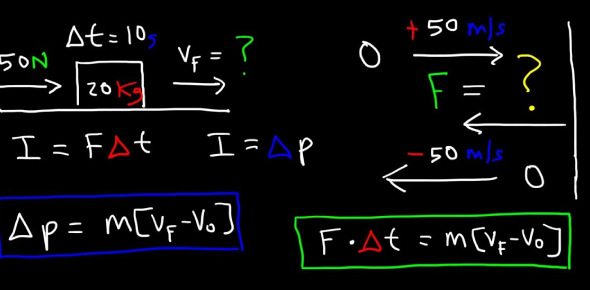# Impulse And Momentum Quiz Under Mr Tan

10 Questions | Total Attempts: 4978SettingsCreate your own QuizThis is just a short quiz that covers topics relating to impulse and momentum. Please answer these questions in real time and do not disconnect your pc from the internet while answering.

• 1.
The momentum of a body is equal to its mass multiplied by its velocity.
• A.

True

• B.

False

• 2.
A ball of mass 3kg is moving to the right with velocity 3m/s and a ball of mass 1kg is moving to the left with velocity 2m/s. we can use the principle of conservation of mass to determine the velocity of the other ball after the collision.
• A.

True

• B.

False

• 3.
What is true about impulse and momentum
• A.

Impulse deals with velocity while momentum deals with change in velocity

• B.

Impulse is mass times velocity while momentum is force times time

• C.

Momentum is the mass of a substance multiplied by the velocity while impulse is just the change of momentum

• D.

Momentum and impulse are unrelated to each other

• 4.
A 71.25 kilogram motorcycle is moving at a speed of 33.75 m/s. What is the momentum of the cycle?
• A.

Equal to 200 kg m/s

• B.

Greater than 200 kg m/s

• C.

Less than 200 kg m/s

• 5.
A small car (mass = 71.25 kilograms), moving at 52.5 m/s, crashes head on with a 285 kilogram truck moving at 33.75 m/s in the opposite direction (negative velocity). If the two cars become intertwined and mangled (inelastic), with what velocity does the wreckage move? (Don't forget direction!)
• A.

A magnitude lesser than 30 m/s moving in the initial direction of the car

• B.

A magnitude lesser than 30m/s moving in the initial direction of the car

• C.

A magnitude lesser than 30 m/s moving in the initial direction of the truck

• D.

A magnitude greater than 30 m/s moving in the initial direction of the truck

• 6.
Because of the principle of conservation of momentum, a rocket can get rid of its empty stages
• A.

To decrease velocity

• B.

To maintain velocity

• C.

To increase mass

• D.

To increase velocity

• 7.
A 50 kg skateboarder is riding his 2.3 kg skateboard at a speed of 4 m/s.What is his momentum?
• A.

Greater than 200 kg m/s

• B.

In between 100 and 200 kg m/s

• C.

Less than 100 kg m/s

• 8.
A 0.2 kg red cue ball rolling at 1.4 m/s elastically collides directly with a 0.2 kg 9-Ball at rest.Which of the following is true?
• A.

The 9-ball would have a velocity greater than 2 m/s

• B.

The 9-ball would have a velocity greater than 0.5 m/s but less than 2m/s

• C.

The red cue ball would have a velocity greater than 1.0 m/s

• D.

The red cue ball would have a velocity of 0 m/s

• E.

The two cue balls would stick together and have the same velocity

• 9.
In a perfectly elastic collision, a ball dropped from a certain height would bounce
• A.

At a greater height

• B.

At the same height

• C.

At a lesser height

• D.

Would stick to the floor

• 10.
In a perfectly inelastic collision, a ball dropped from a certain height would bounce
• A.

At a greater height

• B.

At the same height

• C.

At a lesser height

• D.

Would stick to the floor

Related TopicsBack to top
×

Wait!
Here's an interesting quiz for you.